Mathematics

# Why you need to learn about Percentage to Decimals?

257 views

 1 Introduction 2 Basics of Percentage 3 Simple Percentage 4 Solved Examples 5 Interpreting Percentages 6 Percentage by Ratio 7 Increase or Decrease Percentage 8 Basics of Decimals 9 Representation of Decimal numbers 10 Operations on Decimal Numbers 11 Converting Percentages Into Decimals 12 Converting Decimal Into Percentages 13 Solved Examples 14 Activity 15 Summary 16 About Cuemath 17 Frequently Asked Questions (FAQs) 18 External References

30 September 2020

## Introduction

On a daily basis, we encounter a lot of ideas related to fractions, decimals, ratios, and percentages. Percentages are used widely. They are used as a tool for comparison, used to estimate interest rates in banks, to find profit or loss in business transactions, statistics, and a lot more.

We use decimals every day while dealing with length, weight, money, etc. Decimal numbers are essential when we require precision. Measurement of quantities is done using decimals.

The understanding of these ideas, Percentages, and Decimals are not only essential to being numerate but they are also foundational to topics such as similarity, trigonometry, coordinate geometry, and algebra. So let us see how to use the ideas of Percentages and Decimals and the relationship between them.

Decimals to Fractions to Percentages - The Relationship Between Them

If you ever want to read it again as many times as you want, here is a downloadable PDF to explore more.

## Basics of Percentage

The word ‘percent means ‘out of 100‘. The numerator of a fraction with a denominator of 100 is called percentage.

We can model percentages using a square that has been into 100 small squares. Take the grid below as an example.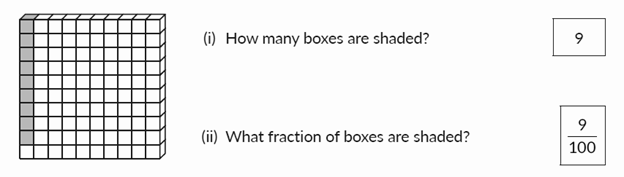There are 100 boxes, 20 are shaded. Then 20% of the boxes are shaded.

In the grid above, what percent of boxes are shaded?

The percentage is also a way of comparing two quantities.

Suppose if Reena got 30 out of 40 in the Maths test and 27 out of 30 in the Science test.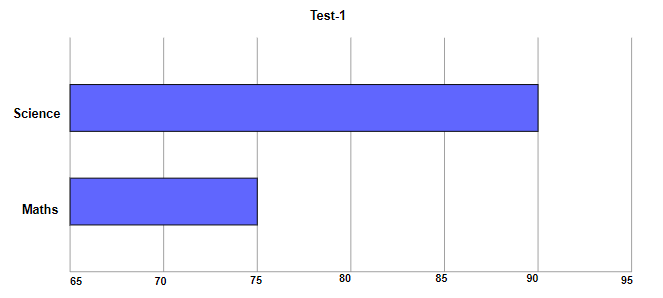In which test did she fare better?

$$\frac{30}{40}$$ in Maths and $$\frac{27}{30}$$ in Science, it is not right to say that she did well in Maths just by seeing the numerators. The maximum marks of both the tests are different. We need to convert the marks in the two tests into percentage and then compare.

Reena’s percentage in Maths $$=\frac{30}{40}\times 100=75\%$$

Reena’s percentage in Science $$=\frac{27}{30}\times 100=90\%$$

So, she fared better in Science.

### Representation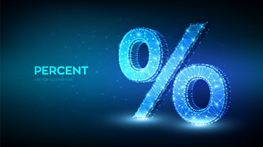A percentage is a symbol. A symbol representing a fraction with a denominator of 100. So, in written language, it is more convenient to write ‘%’ rather than the fraction:

$13\%=\frac{13}{100}$

The symbol (%) is read as ”percent” and indicates the number of parts of a unit, or a reference amount, divided. Therefore, the percentage (%) always appears in an expression that relates two quantities.

## Simple Percentage

Fractions with a denominator of 100 are written merely as percentages.

For example,

$\frac{33}{100}=33\% \quad \frac{175}{100}=175\%$

To write a fraction as a percentage, check if it is possible to find an equivalent fraction with a denominator of 100.

For example,

$$\frac{3}{5}=\frac{3\times20}{5\times20}=\frac{60}{100}=60\% \quad \text{similarly }\frac{3}{2} \frac{150}{100}=150\%$$

When a fraction lacks an equivalent fraction with 100 as the denominator, multiply the fraction by 100%. There is no change to the value of the fraction as 100% = 100/100 = 1.

For example,

$\frac{1}{6}=\frac{1}{6}\times 100\%=\frac{50}{3}=\% =16\frac{2}{3}\%$

Let us see how attendance percentages are calculated for students attending online classes at the school.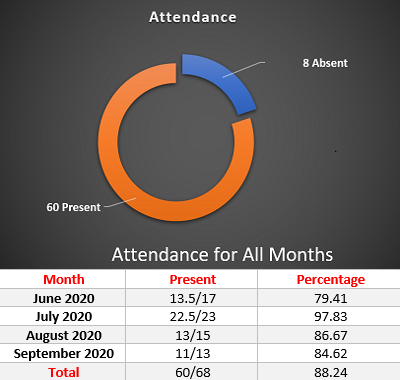The number of days the student attended classes from June-2020 till September-2020 was 60 out of the total number of days 68 as shown in the snapshot. Hence the fraction representing the number of days the student attended classes to the total number of days is  $$\frac{60}{68}$$

Also, Attendance Percentage $$\frac{60}{68}\times100= 88.24\%$$

## Solved Examples

 Example 1

There are 36 fruits in a basket. 9 are mangoes and the remaining are apples. What percentage of fruits are mangoes?

Number of mangoes $$= 9$$

Total number of fruits in a basket $$= 36$$

So, percentage of mangoes $$=\frac{9}{36}\times100=25\%$$

 Example 2

Percentages can be used to compare fractions. In every pair of fractions, write out the greater fraction after converting both fractions to percentages.

$$\frac{12}{75}\times100=16\% \qquad \frac{45}{225}\times100=20\%$$

Greater Fraction $$=\frac{45}{225}$$

## Interpreting Percentages

In math, Percentages are always preferred mostly because they can be converted into fractions and decimals and used in equations.

### Examples

 Example 1

To find eighteen percent of 2,600.

Step 1: Convert sixteen percent into a decimal by moving the decimal point over two places, making 18% into .18

Step 2: The problem would look like this,

$$(.18) × (2,600) = 224.$$ So, eighteen percent of 2,600 is 468.

 Example 2

To find the number whose 20% is 50.

We need to find the number whose 20% or $$\frac{1}{5}th$$ is 50

Here's a strip. 50 is the value of $$\frac{1}{5}th$$ of the whole strip.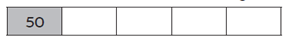Since the strip's been divided into five equal parts, the value of every other part is 50.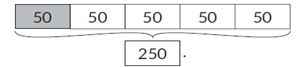This means the value of the strip  is 250.

\begin{align}\text{or, } \frac{1}{5} \text{of }?&=50\\?&=50 \times \frac{5}{1}\\?&=250 \end{align}

 The number is 250

## Percentage by Ratio

Now let us see another way of comparing quantities. We are going to compare the expenditure of two families.

James spends Rs.7040, and Jones spends Rs.7650 every month. Who spends more? As 7650 is more than 7040, can we say that Jones spends more than James? If we compare the expenditure with respect to their monthly income, we will get a better idea of their expenditure.

Suppose if James’s monthly income is Rs.8000 and Jone’s monthly income is Rs.9000, we can compare their expenditure as follows.

The ratio of Jame’s expenditure to his income is 7040 : 8000 = 22:25 = 22/25

The ratio of Jone’s expenditure to his income is 7650 : 9000 = 17:20 = 17/20.

So 22/25 will become 88/100

and 17/20 will be equal to 85/100.

Here we find a fraction equivalent to 22/25 and 17/20. The fractions 88/100, 85/100 are fractions with the same denominator 100. Now it is clear that James spends more than Jones.

### Some more examples

Convert the fractions to percentages.

(a) $$\frac{3}{4}=\frac{3 \times 25}{4\times 25}=\frac{75}{100}=75\%$$

(b)  If the ratio of girls to boys in a class is 9 :11. Find the percentage of boys and girls.

\begin{align}&\text{Percentage of boys }=\frac{11}{11+9}\times 100\%=\frac{11}{11+20}\times100\%=55\%\\&\text{Percentage of girls }=\frac{9}{20}\times 100=45\%\end{align}

## Increase or Decrease Percentage

How do we calculate the change in a quantity as a percentage? It may be necessary to find the difference between the two numbers and identify the increase or decrease percentage. Just like determining percentages, this is a simple process.Another example is that, the price of one litre of oil increases from ₹84 to ₹96. Find the percentage increase in the price of the oil.

Original price = ₹84

Change in price =96 - 84 = ₹12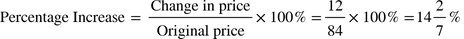And if we add the percentages we get 100%. For instance, in a hostel if 30% of the people are students, 65% are working ladies and 5% are the workers, if we add all the parts of the whole we get 100. So if we are given one part we can always find the other part.

See this example, there is a basket full of mangoes and oranges. If 65% are mangoes, then the percentage of oranges is

(100-65)%=35%

## Basics of Decimals

Fractions with denominators 10,100,1000,10000…are called Decimals. In the decimal number 1.0827, the digits 0, 8, 2 and 7 are called decimal digits. The decimal point goes between Ones and Tenths.

The word decimal comes from the Latin word decem, meaning ‘ten’. The decimal notation uses tenths, hundredths, thousandths, and so on, as well as ones, tens, hundreds, thousands, and so on as shown in the figure below: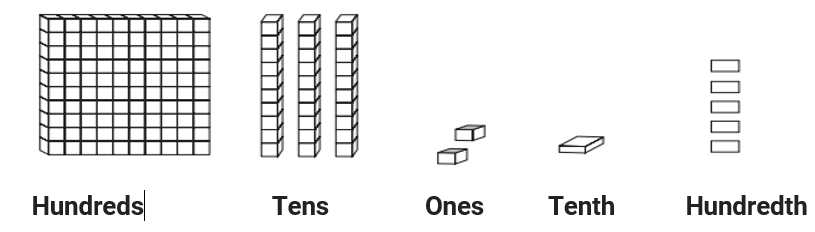Tenths: Place value of the place immediately after the decimal point (i.e.tenth place) is $$\frac{1}{10}$$

Hundredths: The place value of the place directly next to the tenth place is known as the hundredth place $$\frac{1}{100}$$

Place – value expansion of a decimal number

e.g. 253.417

 Hundreds Tens Ones Tenths Hundredths Thousandths Numbers 2 5 3 4 1 7 253.417

## Representation of Decimal numbers

We can represent decimal numbers using Base-10 blocks (flats, rods, and blocks). A flat represents one whole unit, a rod one-tenth of the whole and a block one-hundredth of the whole.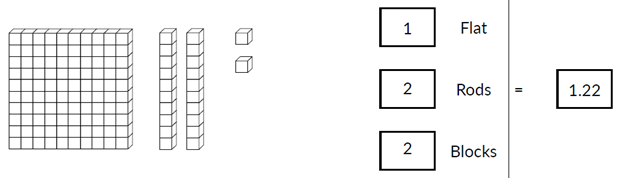Another way of representing decimal numbers is on the abacus.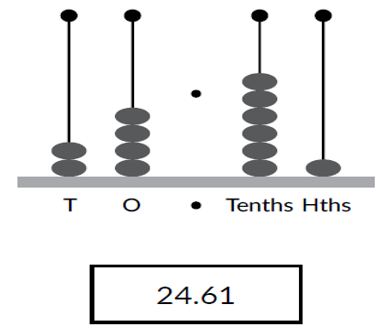## Operations on Decimal Numbers

Addition: 2.3 + 1.2 = 3.5 (ones + ones and tenth + tenth)

Subtract: 2.5 - 1.4 = 1.1 (ones-ones and tenth - tenth)

Multiplication: If multiples of 10 like 10, 100, or 1000 and so on are multiplied by a decimal number, we can obtain the answer by shifting the decimal point to the right the same number of times as the number of zeros. If there are no places left to shift the decimal point, we add zeros after the number.

$$e.g. 0.07 × 10 = 0.7$$

$$0.07 × 100 = 7$$

$$0.07 × 1000 = 70$$

If we want to multiply two decimal numbers, we first multiply the whole number without the decimal point. Then we count the number of digits on the right of the decimal point in both the numbers. Add the number of digits counted. Put the decimal point in the product by counting the number of digits equal to the sum obtained from its rightmost place.

$$e.g. 1.2 × 2.43 = 2.916.$$

Division: While dividing a number by 10, 100, or 1000, the digits of the number and the quotient are the same, but the decimal point in the quotient shifts to the left by as many places as there are zeroes over 1.

$$e.g. 2.38 ÷ 10 = 0.238$$

$$2.38 ÷ 100 = 0.0238$$

$$2.38 ÷ 1000 = 0.00238$$

As a general rule, two decimal numbers are the same only if the fractions they represent are equivalent. This is because adding a zero to the right of a decimal number is the same as multiplying its fractional representation by 10 / 10.

For example,

$$\frac{3}{10}=0.3$$
and $$\frac{3 \times 10}{10 \times 10}=\frac{30}{100}=0.30$$
and $$\frac{3 \times 10 \times 10}{10 \times 10 \times 10}=\frac{300}{1000}=0,300$$

which means that 0.3 = 0.30 = 0.300 and so on.

And this is why we say that "adding zeros to the right of a decimal number does not change its value.

## Converting Percentages Into Decimals

Let us see how to carry out the Percentage to Decimal conversion.

2735/100 is the fractional form of the decimal number 27.35.

For percentage to decimal conversion, we have to divide the percentage by 100 and remove the percentage(%) sign.

For example $$76\%=\frac{76}{100}=0.76$$

‘Whole number’ percentages can easily be written as decimals by writing them as fractions with a denominator of 100 and converting to a decimal. For example,$$65\% = 65 100 = 0.65 \quad 150\% = \frac{150}{100} = \frac{15}{10} = 1.5.$$

To write a percentage as a decimal we divide by 100.

For example, $$62.5\% = \frac{62.5}{100} = 0.625$$

## Converting Decimal Into Percentages

For decimal to percentage conversion, simply multiply by 100. For example,

$$0.77=0.77\times100\%=77%$$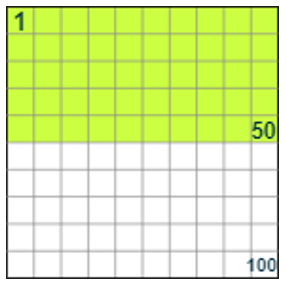These all mean the same thing:

• 0.5 (half) of the box is green.
• 50 of the 100 squares are green,
• $$\frac{50}{100}$$ of the box is green,
• 50% of the box is green.

The following flow diagram depicts decimal to percentage conversion and vice versa.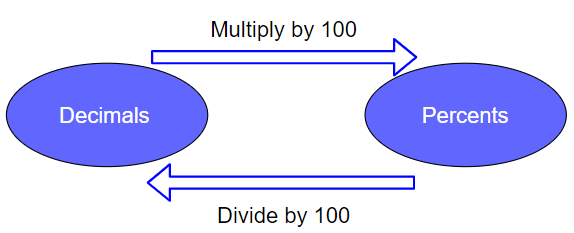## Solved Examples

 Example 1

Convert each of the following percentages to decimals

$$60\% =\frac{60}{100}$$ Remove percent symbol and divide by 100

 $$= 0.6$$
 Example 2

Convert each of the following decimals to percentages

$$0.04 = 0.04 × 100$$ Multiply by 100 and add % symbol

 $$= 4\%$$

Not all percentages can be expressed as fractions and figures such as 3.26% may need to be used as shown in the given example.

 Example 3

The cost of a hotel bill is £200. VAT at 17.5% has to be added to this bill. Find the VAT and the total bill.

Solution

Here 17.5% can be written as 0.175

So 17.5% of £200 = 0.175 × 200

VAT = £35

 Total bill is now = £200 + £35 =£235.

The following table shows the relation between Fractions, Percentage,s and Decimals.

 Fraction Decimal Percentage $$\frac{1}{10}$$ 0.1 10% $$\frac{1}{5}$$ 0.2 20% $$\frac{3}{10}$$ 0.3 30% $$\frac{2}{5}$$ 0.4 40% $$\frac{1}{2}$$ 0.5 50% $$\frac{3}{5}$$ 0.6 60% $$\frac{7}{10}$$ 0.7 70% $$\frac{4}{5}$$ 0.8 80% $$\frac{9}{10}$$ 0.9 90% $$\frac{1}{4}$$ 0.25 25% $$\frac{3}{4}$$ 0.75 75%

Table relating Fractions, Decimals and Percentage

From the above table we can understand that the relationship between decimals and percents is that they are merely different numerical expressions of the same value.

## Activity

Try This: That’s 100 %

### Materials Required

1. Fraction strips
2. A4 size chart sheets (3 numbers each of different colour)
• This activity gives a comfortable technique to evaluate a value based on the given percentage.
• To calculate values based on percentage create a simple easy percentage bar model. This model shows the fraction and also the decimal equivalent to the respective percentages.
• Consider the problem. If 30% equals 24, which is the number of girls in a class then what is the total strength? It’s very easy to calculate using this model.
• For 30% the fraction value is 3/10 and its reciprocal is 10/3. The answer is 10/3 of 24 and its equal to 80.
• Similarly, if 35% of 60 is to be calculated then it’s just 0.35 X 60 = 21.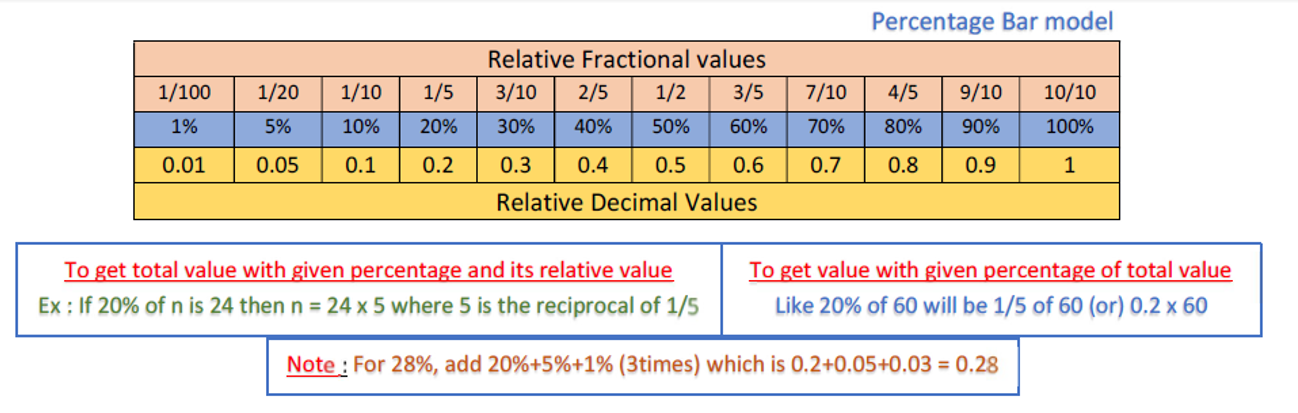## Summary

### Real-life Usage of Percentage and Decimals

Percentages play a vital role in the evaluation of marks, understanding the growth of the market value for any product with respect to a given scenario, in banks for calculating interests based on different kinds of deposits.

Decimals are used widely. In our daily life, we use decimals while dealing with money, weight, and length.

Online store checking provides lots of opportunities for children to explore percentages and decimals. We could ask questions such as

• How much should we pay if we buy 3 boxes of 10 chocolates that cost Rs.2.60 each?
• What is the new price of an Rs1800 MI Super Bass headset if there is a 10% discount?

This concept has a profound impact on everyday life in numerous professions and is extremely important. This article covered all the basic concepts with examples.

Cuemath, a student-friendly mathematics platform, conducts regular Online Live Classes for academics and skill-development, and their Mental Math App, on both iOS and Android, is a one-stop solution for kids to develop multiple skills. Understand the Cuemath Fee structure and sign up for a free trial.

## What is the history of Percentages?

The history of Percentages dates back to the Roman period. Roman taxes were calculated on the basis of percentages. For example, Augustus levied a tax of 1% on goods sold at auction and there was a tax of 4% on every slave sold. The Romans did not use this notation but certainly employed the concept that we use today.

## Why is a gallon in the UK 20% bigger than a gallon in the USA?

In the USA, a gallon is equivalent to 128 fluid ounces or 3.785 liters. But in the UK, it's 160 fluid ounces or 4.546 liters. That's quite a difference, with the British contenders having to potentially guzzle down 20% more milk than their American counterparts.

## What is the history of Decimals?

Decimal fractions had been employed for the extraction of square roots by Islamic mathematicians such as Al-Kashi (1380 – 1429) and also by the Chinese at an even earlier time. The introduction of decimal fractions as a common computational practice can be dated back to the Flemish pamphlet De Thiede published at Leyden in 1585, together with a French translation, La Disme, by the Flemish mathematician Simon Stevin (1548-1620). Will need a new similar image.